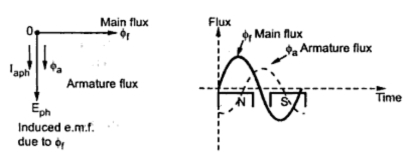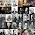Armature Reaction

When the load is connected to the alternator, the armature winding of the alternator carries a current. Every current carrying conductor produces its own flux so armature of the alternator also produces its own flux, when carrying a current. So there are two fluxes present in the air gap, one due to armature current while second is produced by the filed winding called main flux. The flux produced by the armature is called armature flux.
Note : So effect of the armature flux on the main flux affecting its value and the distribution is called armature reaction.
The effect of the armature flux not only depends on the magnitude of the current flowing through the armature winding but also depends on the nature of the power factor of the load connected to the alternator.
Now we will study the effect of nature of the load power factor on the armature reaction.
1.1  Unity Power Factor Load
Consider a purely resistive load connected to the alternator, having unity power factor. As induced e.m.f. Eph drives a current of Iaph  and load power factor is unity, Eph and Iph  are in phase with each other.
If Φ is the main flux produced by the field winding responsible for producing Eph then Eph lags Φ by 90o .
Now current through armature Ia, produces the armature flux say Φa. So flux Φa and Ia are always in the same direction.
This relation between Φf , Φa, Eph and Iaph  can be shown in the phasor diagram. (See Fig. 1)Fig. 1  Armature reaction for unity power factor
It can be seen from the phasor diagram that there exists a phase difference of  90o between the armature flux and the main flux. The waveforms for the two fluxes are also shown in the Fig. 1. From the waveforms it can be seen that the two fluxes oppose each other on the left half of each pole while assist each other on the right half of each pole. Hence average flux in the air gap remains constant but its distribution gets distrorted.
Note : Hence such distorting effect of armature reaction under unity p.f. condition of the load is called cross magnetising effect of armature reaction.
Due to such distortion of the flux, there is small drop in the terminal voltage of the alternator.
1.2 Zero Lagging Power Factor Load
Consider a purely inductive load connected to the alternator having zero lagging power factor. This indicates that Iaph  driven by Eph lags Eph by 90o which is the power factor angle Φ.
Induced e.m.f. Eph lags main flux Φf by 90o while Φa is in the same direction as that of  Ia. So the phasor diagram and the waveforms are shown in the Fig. 2.
It can be seen from the phasor diagram that the armature flux and the main flux are exactly in opposite direction to each other.
Note : So armature flux tries to cancel the main flux. Such an effect of armature reaction is called demagnetising effect of the armature reaction.
As this effect causes reduction in the main flux, the terminal voltage drops. This drop in the terminal voltage is more than the drop corresponding to the unity p.f. load.Fig. 2  Armature reaction for zero lagging p.f. load
Consider a purely capacitive load connected to the alternator having zero leading power factor. This means that armature current Iaph  driven by Eph, leads Eph by 90o, which is the power factor angle Φ.
Induced e.m.f. Eph lags Φf by 90o while Iaph  and Φa are always in the same direction. The phasor diagram and the waveforms are shown in the Fig. 3.Fig. 3 Armature reaction for zero leading p.f. load
It can be seen from the phasor diagram and waveforms shown in the Fig. 2, the armature flux and the main field flux are in the same direction i.e. they are helping each other. This results into the addition in main flux.
Note : Such an effect of armature reaction due to which armature flux assists field flux is called magnetising effect of the armature reaction.
As this effect adds the flux to the main flux, greater e.m.f. gets induced in the armature. Hence there is increase in the terminal voltage for leading power factor loads.
For intermediate power factor loads i.e. between zero lagging and zero leading the armature reaction is partly cross magnetising and partly demagnetising for lagging power factor loads or partly magnetising for leading power factor loads.
1.4 Armature Reaction Reactance (Xar)
In all the conditions of the load power factors, there is change in the terminal voltage due to the armature reaction. Mainly the practical loads are inductive in nature, due to demagnetising effect of armature reaction, there is reduction in the terminal voltage. Now this drop in the voltage due to the interaction of armature and main flux. This drop is not across any physical element.
But to quantify the voltage drop due to the armature reaction, armature winding is assumed to have a fictitious reactance. This fictitious reactance of the armature is called armature reaction reactance denoted as  Xar Ω/ph. And the drop due to armature reaction can be accounted as the voltage drop across this reactance as Iar Xar.
Note : The value of this reactance changes as the load power factor changes, as armature reaction depends on the load power factor.

1 comment:

1.Hi,
sir can we say Xar is capacitive reactance in case of purely capacitive load. Or Xar ha '-' minus sign in case of capacitive load?